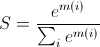## Tuesday, August 23, 2011

### Avoiding overflow problem in softmax and alike expressions

While underflow is a common problem in probabilistic machine learning structures like HMMs, overflow problems are common in neural network-like structures.
In the case of overflow in an expression of type:it may happen that either f(i) or the denominator overflows.
A possible solution is to put the expression in a softmax style form like:and use a trick for this expression, which says that you can rewrite the expression as:where you can choose any K. With a correct K this will make sure that the exponentials don't blow up causing overflow.

Now we know this, all you have to notice is that we can writeand put it into your initial expression to have it in the softmax form and use the trick.

Still, we need to select K. Although I don't know about any sophisticated method, what works for me is to set K=max( m(i)) or similarly K= max( log(f(i))). This will make sure that the biggest m(i) does not overflow. Of course, this might cause smaller m(i)s to underflow, but their contribution would have been negligible anyway.

One place where this worked for me is while implementing a Discriminative RBM (DRBM) as in . Where you can see that p(y|x) has the form mentioned here.